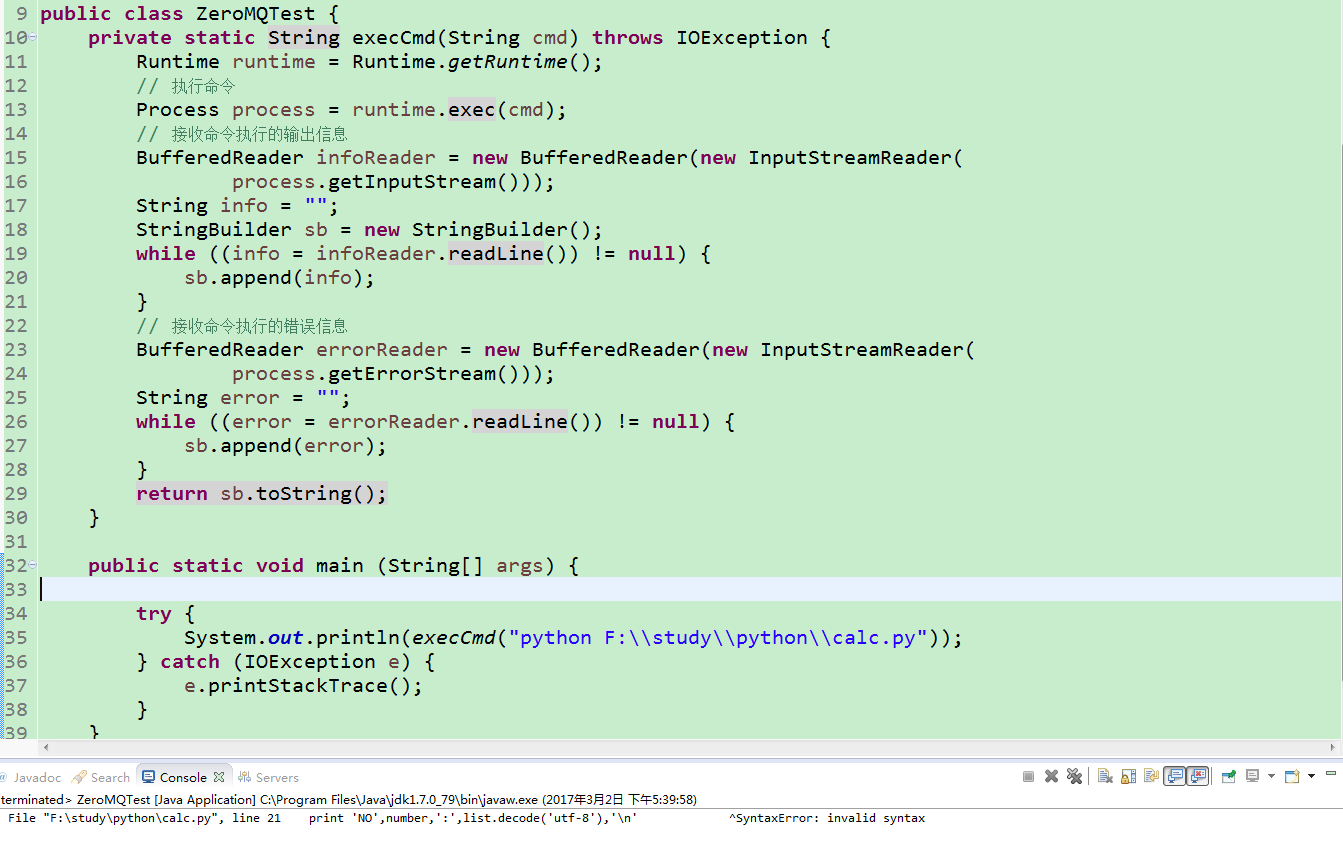## Eclipse中java代码调用python脚本时提示错误Exception in thread "main" Traceback (most recent call last):，请问如何解决？

java代码如下：

``````package test;

import org.python.util.PythonInterpreter;

public class ThirdJavaScript {
public static void main(String args[]) {
PythonInterpreter interpreter = new PythonInterpreter();
interpreter.execfile("E:\\python\\python_demo\\see_xiaohua.py");
}
}``````

``````# coding:utf-8
#!/usr/bin/python
import urllib2#与下面脚本对比没有被注释
import re#与下面脚本对比没有被注释
from bs4 import BeautifulSoup#与下面脚本对比没有被注释
number =0  #定义序号变量
page = raw_input("Please input page:")
user_agent = 'Mozilla/4.0 (compatible; MSIE 5.5; Windows NT)'
headers = { 'User-Agent' : user_agent }
response = urllib2.urlopen(request)
html = response.read()   #获取页面源码
soup = BeautifulSoup(html,"html.parser")
items = soup.find_all('div',attrs={"class":"content"}) #搜索div标签并且同时含有class=content内容

for item in items:
number +=1
pattern = re.compile('<span>(.*?)</span>',re.S)
lists = re.findall(pattern,str(item))
for list in lists:
print 'NO',number,':',list.decode('utf-8'),'\n'

print "End..."
``````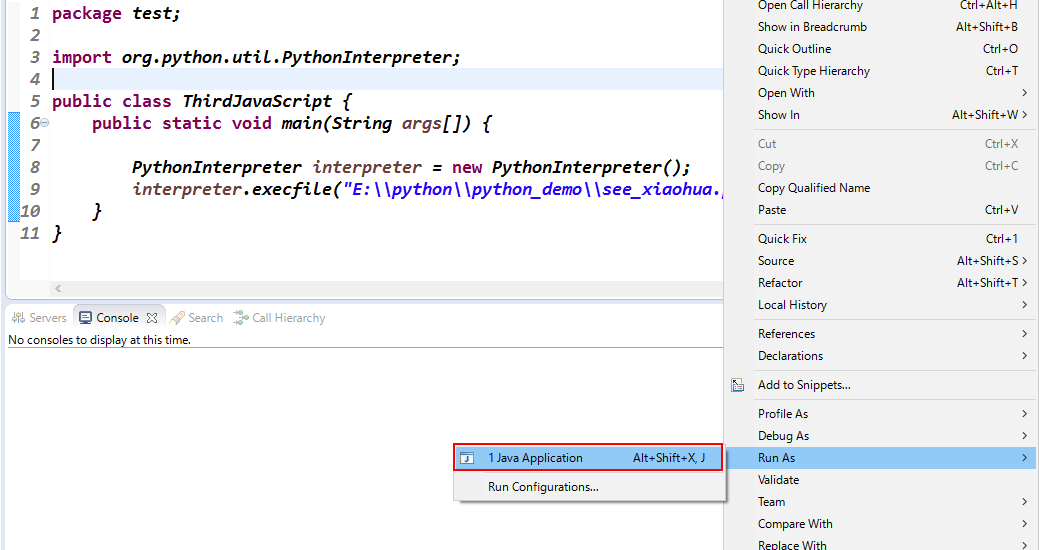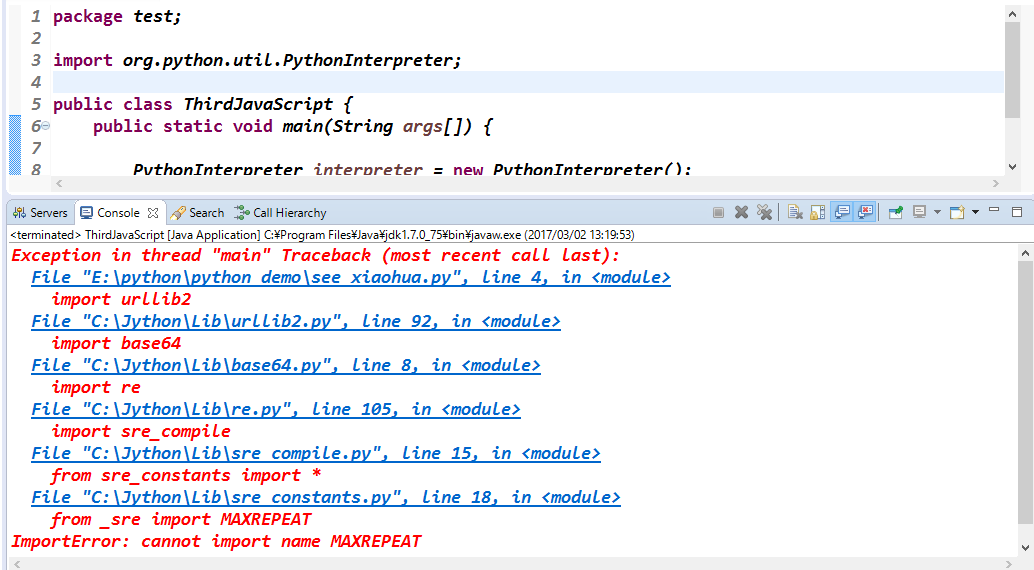0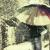0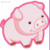#### 引用来自“Ginray”的评论

0#### 引用来自“非著名魔兽解说”的评论

0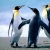``````/**
* 命令执行器
* @param cmd
* @return
* @throws IOException
*/
private static String execCmd(String cmd) throws IOException{
LOGGER.info("execCmd: cmd ---> " + cmd);
Runtime runtime = Runtime.getRuntime();
//执行命令
Process process = runtime.exec(cmd);
//接收命令执行的输出信息
String info = "";
StringBuilder sb = new StringBuilder();
LOGGER.info(info);
sb.append(info);
}
//接收命令执行的错误信息
String error = "";
LOGGER.error(error);
sb.append(error);
}
LOGGER.info("execCmd end.");
return sb.toString();
}
``````

0#### 引用来自“gitlab_sam”的评论

``````package test;

import java.io.*;

public class ThirdJavaScript {
public static void main(String args[]) {

// PythonInterpreter interpreter = new PythonInterpreter();
// interpreter.execfile("E:\\python\\python_demo\\inputpy.py");
// interpreter.execfile("E:\\python\\python_demo\\see_xiaohua.py");
try {
execCmd("python E:\\python\\python_demo\\see_xiaohua.py");
} catch (IOException e) {
e.printStackTrace();
}
}

private static String execCmd(String cmd) throws IOException {
Runtime runtime = Runtime.getRuntime();
// 执行命令
Process process = runtime.exec(cmd);
// 接收命令执行的输出信息
process.getInputStream()));
String info = "";
StringBuilder sb = new StringBuilder();
while ((info = infoReader.readLine()) != null) {
sb.append(info);
}
// 接收命令执行的错误信息
process.getErrorStream()));
String error = "";
while ((error = errorReader.readLine()) != null) {
sb.append(error);
}
return sb.toString();
}
}
``````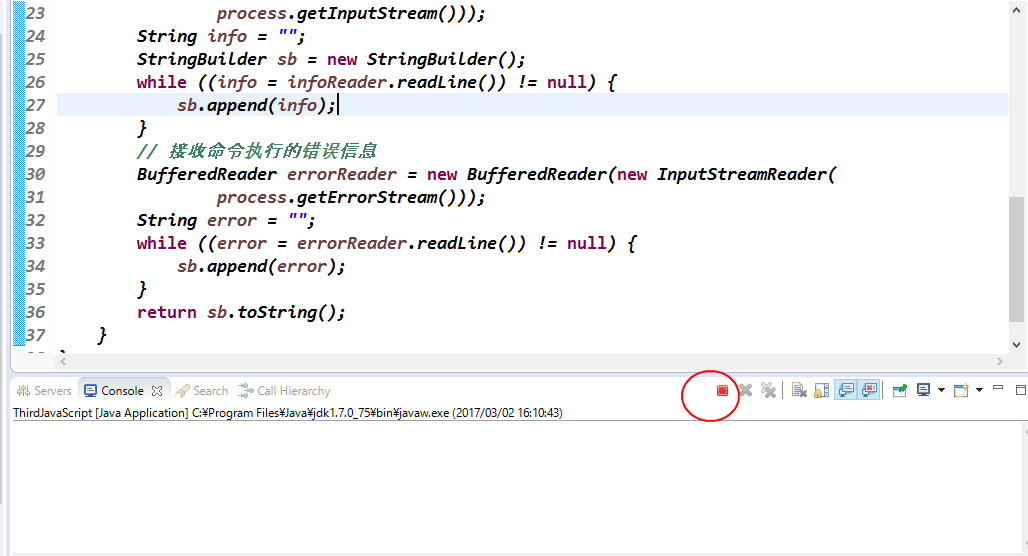0#### 引用来自“gitlab_sam”的评论

``````package test;

import java.io.*;

public class ThirdJavaScript {
public static void main(String args[]) {

// PythonInterpreter interpreter = new PythonInterpreter();
// interpreter.execfile("E:\\python\\python_demo\\inputpy.py");
// interpreter.execfile("E:\\python\\python_demo\\see_xiaohua.py");
try {
execCmd("python E:\\python\\python_demo\\see_xiaohua.py");
} catch (IOException e) {
e.printStackTrace();
}
}

private static String execCmd(String cmd) throws IOException {
Runtime runtime = Runtime.getRuntime();
// 执行命令
Process process = runtime.exec(cmd);
// 接收命令执行的输出信息
process.getInputStream()));
String info = "";
StringBuilder sb = new StringBuilder();
while ((info = infoReader.readLine()) != null) {
sb.append(info);
}
// 接收命令执行的错误信息
process.getErrorStream()));
String error = "";
while ((error = errorReader.readLine()) != null) {
sb.append(error);
}
return sb.toString();
}
}
``````0#### 引用来自“gitlab_sam”的评论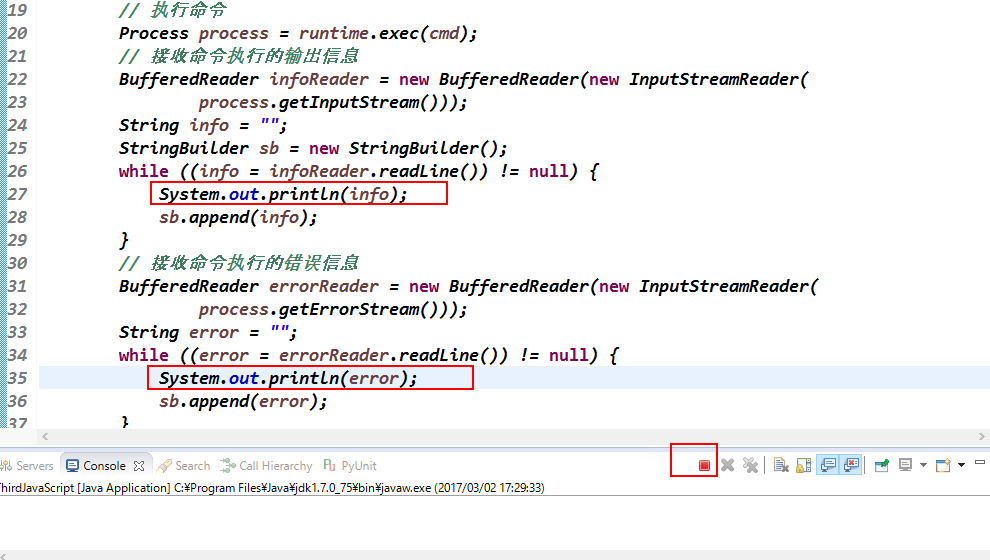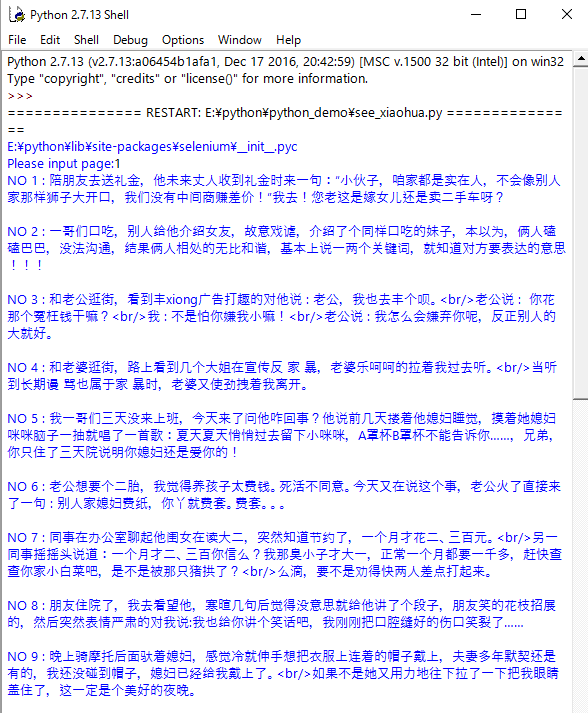0#### 引用来自“gitlab_sam”的评论